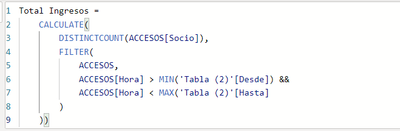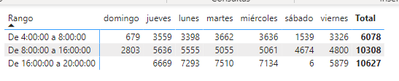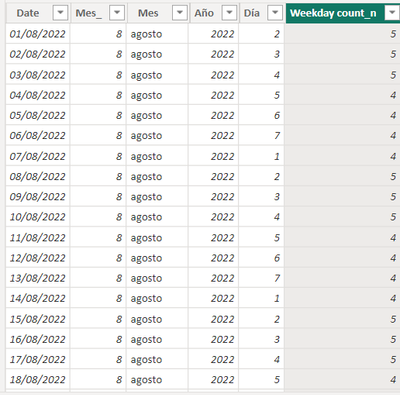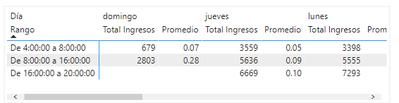cancel
Showing results for
Did you mean:Frequent Visitor

## Promedio de asistencias

Hola, tengo una tabla de asistencias con fechas de diferentes meses que incluye el código de cada socios que ingresa, la fecha y la hora, y luce algo así:Me interesa conocer las horas pico donde ingresa la mayor cantidad de socios, para ello dividí el día laboral en 3:Utilicé el siguiente comando para poder observar cuántos socios ingresan por día en cada uno de los 3 horarios (ojo, un socio puede ingresar más de una vez en un día, o ingresar, salir y volver a ingresar en el mismo momento, por lo que me interesa que el conteo de los socios sea distintivo):Y esto es lo que me arroja dicho comando:Ahora que tengo esto, estoy interesado en conocer el promedio de ingresos por día, por ejemplo, el jueves ingresaron 3559 socios de 4 am a 8 am, suponiendo que dicho mes hubo 5 jueves, el promedio sería: 3559/5 = 772, pero no sé cómo hacerlo, lo intenté con Averagex pero me da resultados erróneos, o tal vez estoy aplicando mal el comando. Quisiera poder agregar dicha medida a la tabla que está arriba, en el ejemplo dado poder ver:

Jueves

Total      Promedio
4 am - 8 am      3559           772
¿Alguna idea para poder hacer esto? Desde ya muchas gracias por el apoyo.

6 REPLIES 6Community Support

Hi @jsama ,

Create a calendar table.

``table=calendar(date(2020,1,1),date(2022,12,31))``

Then create columns.

``month=month(table[date])``
``Year=year(table[date])``
``weekday=weekday(table[date])``
``````weekday count =
CALCULATE (
COUNT ( table[weekday] ),
FILTER (
table,
table[month] = EARLIER ( table[month] )
&& table[year] = EARLIER ( table[year] )
&& table[weekday] = EARLIER ( table[weekday] )
)
)
``````

Then create relationships between the tables.

Then create a measure.

``measure=[total Ingresos]/max(table[weekday count])``

If it does not help, please provide more details with your desired output and pbix file without privacy information (or some sample data) .

Best Regards
Community Support Team _ Polly

If this post helps, then please consider Accept it as the solution to help the other members find it more quickly.Frequent Visitor

I just have a final problem, I did what you told me, and it helped me a lot, but when I use the measure

``measure=[total Ingresos]/max(table[weekday count])``

It always gives me the max number of the month, and thats not always right, let me give you an example:

In August 2022 there where 4 sundays, so when I divide the total of access it should be: 307/4 = 76.7, but the Max number of the column "weekday count" its 5, it divides the total of access by 5. If there were 4 sundays I need to divide the total of access by 4.Community Support

Hi @jsama ,

Change the weekday column.

``weekday=weekday(table[date],2)``
``````weekday count =
CALCULATE (
COUNT ( table[weekday] ),
FILTER (
table,
table[month] = EARLIER ( table[month] )
&& table[year] = EARLIER ( table[year] )
&& table[weekday] = 7
)
)``````

Best Regards
Community Support Team _ Polly

If this post helps, then please consider Accept it as the solution to help the other members find it more quickly.Super User

Is the matrix visual you are showing  being filterd for August in one specific year? If so, it should work.

Can you share the pbix?Please accept the solution when done and consider giving a thumbs up if posts are helpful.  Contact me privately for support with any larger-scale BI needs, tutoring, etc.Super User

Hi @jsama

You already have the "Total" so you only need to count the number of "jueves".  What field are you using for the day? A COUNT of that field should suffice. Something like

``COUNT(DateT[WeekDay])``

Then you can divide the total by this valuePlease accept the solution when done and consider giving a thumbs up if posts are helpful.  Contact me privately for support with any larger-scale BI needs, tutoring, etc.Frequent Visitor

I created a column for the field day using:

``Day = format(Date, "dddd")``

I tried with

``count(Date[Day])``

But it gives me the total amount of rows that I have, so when I divide the total by this new value it shows me this:This is the total access of an specific day divided by the total of access.

In the example that I gave, I need the total access of thursday divided by the total of thursday in a period of time. For example, in august there were 4 thursdays, and the total access in august was 3559, so its going to be 3559/4 =  890Announcements#### Exclusive opportunity for Women!

Join us for a free, hands-on Microsoft workshop led by women trainers for women where you will learn how to build a Dashboard in a Day!#### Power Platform Conference-Power BI and Fabric Sessions

Join us Oct 1 - 6 in Las Vegas for the Microsoft Power Platform Conference.Top Solution Authors
Top Kudoed Authors
Users online (1,950)